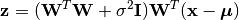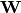Probabilistic Principal Component Analysis¶

Probabilistic Principal Component Analysis (PPCA) represents a constrained form of the Gaussian distribution in which the number of free parameters can be restricted while still allowing the model to capture the dominant correlations in a data set. It is expressed as the maximum likelihood solution of a probabilistic latent variable model [BSHP06].

This package defines a PPCA type to represent a probabilistic PCA model, and provides a set of methods to access the properties.

Properties¶

Let M be an instance of PPCA, d be the dimension of observations, and p be the output dimension (i.e the dimension of the principal subspace)

indim(M)

Get the input dimension d, i.e the dimension of the observation space.

outdim(M)

Get the output dimension p, i.e the dimension of the principal subspace.

mean(M)

Get the mean vector (of length d).

projection(M)

Get the projection matrix (of size (d, p)). Each column of the projection matrix corresponds to a principal component.

The principal components are arranged in descending order of the corresponding variances.

var(M)

The total residual variance.

Transformation and Construction¶

Given a probabilistic PCA model M, one can use it to transform observations into latent variables, asor use it to reconstruct (approximately) the observations from latent variables, asHere,is the factor loadings or weight matrix.

The package provides methods to do so:

transform(M, x)

Transform observations x into latent variables.

Here, x can be either a vector of length d or a matrix where each column is an observation.

reconstruct(M, z)

Approximately reconstruct observations from the latent variable given in z.

Here, y can be either a vector of length p or a matrix where each column gives the latent variables for an observation.

Data Analysis¶

One can use the fit method to perform PCA over a given dataset.

fit(PPCA, X; ...)

Perform probabilistic PCA over the data given in a matrix X. Each column of X is an observation.

This method returns an instance of PCA.

Keyword arguments:

Let (d, n) = size(X) be respectively the input dimension and the number of observations:

name description default
method

The choice of methods:

• :ml: use maximum likelihood version of probabilistic PCA
• :em: use EM version of probabilistic PCA
• :bayes: use Bayesian PCA
:ml
maxoutdim Maximum output dimension. d-1
mean

The mean vector, which can be either of:

• 0: the input data has already been centralized
• nothing: this function will compute the mean
• a pre-computed mean vector
nothing
tol Convergence tolerance 1.0e-6
tot Maximum number of iterations 1000

Notes:

• This function calls ppcaml, ppcaem or bayespca internally, depending on the choice of method.

Example:

using MultivariateStats

# suppose Xtr and Xte are training and testing data matrix,
# with each observation in a column

# train a PCA model
M = fit(PPCA, Xtr; maxoutdim=100)

# apply PCA model to testing set
Yte = transform(M, Xte)

# reconstruct testing observations (approximately)
Xr = reconstruct(M, Yte)

Core Algorithms¶

Three algorithms are implemented in this package: ppcaml, ppcaem, and bayespca.

ppcaml(Z, mean, tw; ...)

Compute probabilistic PCA using on maximum likelihood formulation for a centralized sample matrix Z.

Parameters: Z – provides centralized samples. mean – The mean vector of the original samples, which can be a vector of length d, or an empty vector Float64[] indicating a zero mean. The resultant PPCA model. This function accepts two keyword arguments: maxoutdim and tol.
ppcaem(S, mean, n; ...)

Compute probabilistic PCA based on expectation-maximization algorithm for a given sample covariance matrix S.

Parameters: S – The sample covariance matrix. mean – The mean vector of original samples, which can be a vector of length d, or an empty vector Float64[] indicating a zero mean. n – The number of observations. The resultant PPCA model. This function accepts two keyword arguments: maxoutdim, tol, and tot.
bayespca(S, mean, n; ...)

Compute probabilistic PCA based on Bayesian algorithm for a given sample covariance matrix S.

Parameters: S – The sample covariance matrix. mean – The mean vector of original samples, which can be a vector of length d, or an empty vector Float64[] indicating a zero mean. n – The number of observations. The resultant PPCA model. This function accepts two keyword arguments: maxoutdim, tol, and tot.